# Evaluate the following indefinite integral: e VT -dz C

Evaluate the following indefinite integral: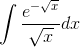e VT -dz C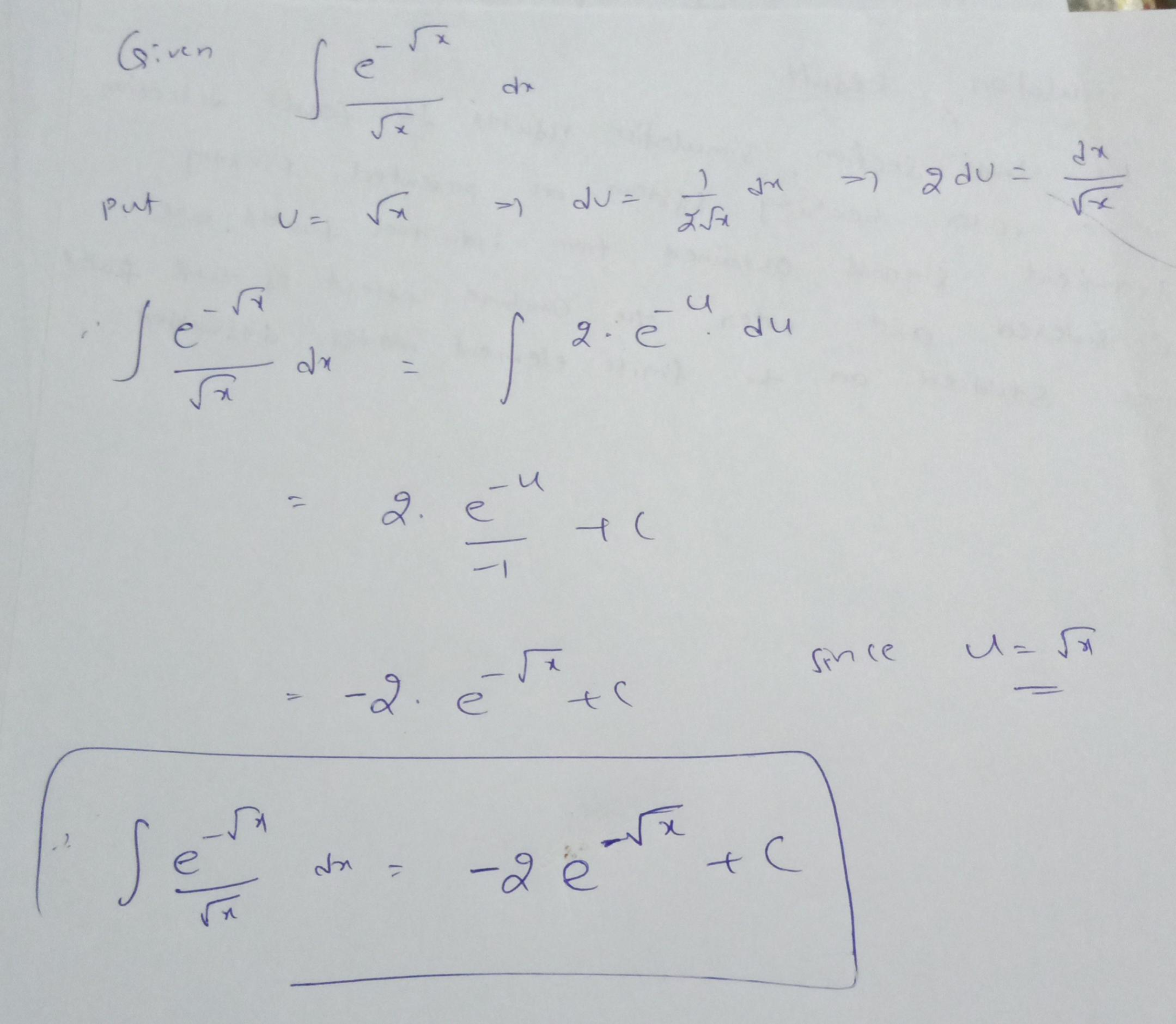##### Add Answer of: Evaluate the following indefinite integral: e VT -dz C
Similar Homework Help Questions
• ### Use Integration by Parts for Indefinite Integrals Question Evaluate In(x) dz Remember to include +C in your answer. Use Integration by Parts for Indefinite Integrals Question Evaluate In(x)...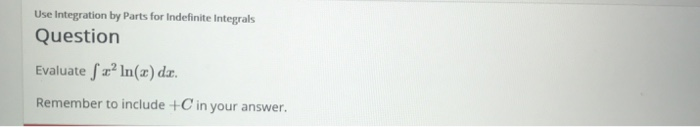Use Integration by Parts for Indefinite Integrals Question Evaluate In(x) dz Remember to include +C in your answer. Use Integration by Parts for Indefinite Integrals Question Evaluate In(x) dz Remember to include +C in your answer.

• ### 1. Use integration by parts to evaluate the integral: ∫ 6z cos(5z) dz Use integration by parts to evaluate the definite integral. 5t2 In tdt Use integration by parts to evaluate the definite integ...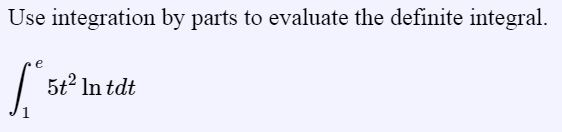1. Use integration by parts to evaluate the integral: ∫ 6z cos(5z) dz Use integration by parts to evaluate the definite integral. 5t2 In tdt Use integration by parts to evaluate the definite integral: 5se3ds J0.2 Preview Report answer accurate to 3 decimal places. A particle that moves along a straight line has velocity v(t)e3 meters per second after t seconds. How many meters will it travel during the first t seconds (from time-0 to time-t)? 2-3t Evaluate the indefinite...

• ### Evaluate the following indefinite integral. (10dx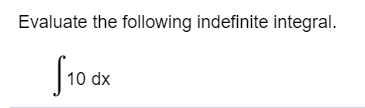Evaluate the following indefinite integral. (10dx

• ### 6. (15points) Evaluate the triple integral pln(y+1) e -2.c *dx dy dz.6. (15points) Evaluate the triple integral pln(y+1) e -2.c *dx dy dz.

• ### Evaluate the following indefinite integral

Evaluate the following indefinite integral. Use C as the arbitrary constant.佺(12 /併x+12併x)dxOnly the x's are under the root symbols.I got 6x^1/2 +8x^3/2+C as my answer. Is that correct?

• ### Evaluate the indefinite integral

Evaluate the indefinite integral.

• ### Evaluate the indefinite integral.Evaluate the indefinite integral.

• ### Evaluate the indefinite integral.Evaluate the indefinite integral.

• ### Evaluate the indefinite integral

Evaluate the indefinite integral. (Use theta for 兤.) 佺(8兤 + cos(7兤))d兤 Ans: blank + CC is constant. How do I evaluate it?

• ### evaluate the indefinite integralEvaluate the given indefinite integral.

Free Homework App